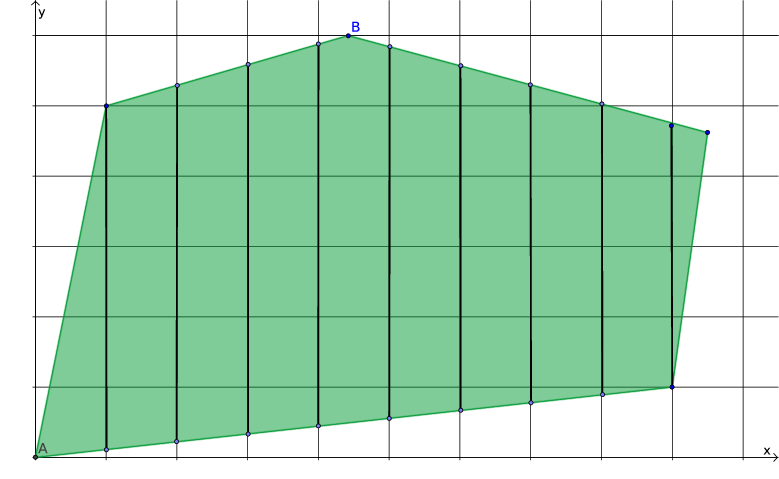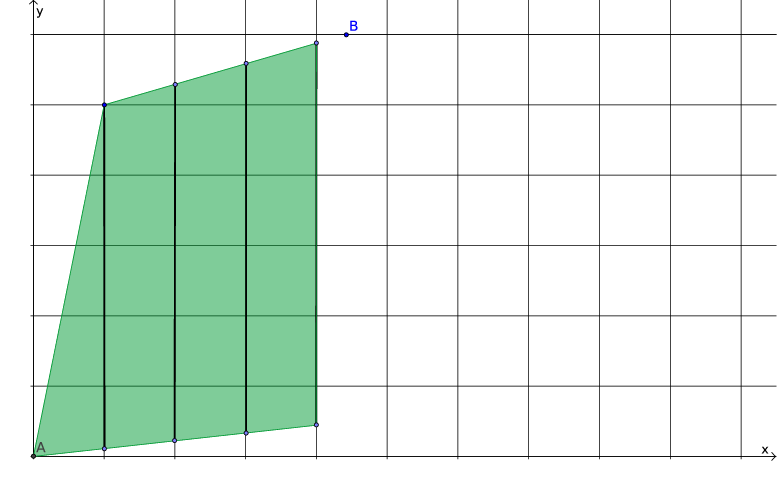## Saturday, February 21, 2015

### Branching Partitions the Feasible Region

Yesterday's post got me started on the subject of the geometry of a linear program (LP). Today I want to review another well-known geometric aspect, this time of integer linear programs (ILPs) and mixed integer linear programs (MILPs), that perhaps slips some people's minds when they are wrestling with their models. Most solvers use some variant of the branch and bound (or, more generally, branch and cut) algorithm.

The point I want to make is in the title of this post: branching (more precisely, separating a node in the branch and bound search tree into child nodes) equates to partitioning the feasible region into disjoint chunks. The interpretation of "feasible region" in that last sentence is a bit tricky. On the one hand, the region being partitioned continues to relax the integrality restriction on at least some of the integer variables, adding points not belonging to the original feasible region. On the other hand, outside of the root node, every node problem restricts variables to a subset of the original feasible region. The problem solved at each node is a linear program (LP), sometimes called the node LP. The feasible region of the node LP at any node other than the root is neither a subset nor a superset of the original feasible region; it's a bit of both.

To clarify that (I hope), consider the two variable MILP illustrated in Figure 1, in which $x$ is a general integer variable and $y$ is a continuous variable.Figure 1: MILP feasible region

The feasible region consists of a bunch of disjoint line segments (integer abscissas $x$, arbitrary ordinates $y$), along with an isolated point (labeled A). If we relax the integrality restriction on $x$, we get the convex polytope shown in Figure 2, which is the feasible region of the LP at the root node.Figure 2: LP relaxation

Now suppose that we are looking for the point that maximizes $y$. The optimal solution to the root LP is labeled B in Figure 2. Let's say that $B=(x_0, y_0)$. Note that the value $x_0$ of $x$ at B is not integral.

One possible way to partition the root node is to round the value of $x$ at B up in one child node and down in the other. In effect, this adds the new constraint (cut) $x\le \left\lfloor x_{0}\right\rfloor$ to one child node (call it the left child), and the cut $x \ge \left\lceil x_{0}\right\rceil$ to the other (right) child. Figures 3 and 4 show the child nodes.Figure 3: Left childFigure 4: Right child
I left point B in both figures as a point of reference, but it does not belong to either feasible region.

In this particular (rather trivial) example, the maximum value of $y$ in both children occurs at an integer-feasible point (a corner where $x$ happens to take an integer value), so there will be no further branching. More generally, the algorithm might choose to split either child into either smaller chunks, etc.

One of the questions I saw not long ago asked why a particular solver would branch twice on the same variable. If that variable were binary, it would not make sense. If $z$ is a binary variable that takes a fractional value (say $z = 0.234$) in the solution of a node LP, the cuts $z \le \left\lfloor 0.234\right\rfloor$ and $z \ge \left\lceil 0.234\right\rceil$ equate to $z=0$ and $z=1$, and there is no way to further reduce the domain of $z$. For a general integer variable, such as $x$ in the illustration above, there may be reason to further subdivide the domain of $x$.

My apologies if this all seems quite basic; it is building up to the next post, where I hope to answer a not entirely trivial question.

1.1.